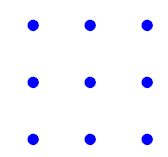# Combinatorics...

here is a 3 x 3 square....how many triangles can be formed with the dots being the three vertices of the triangle??

•Soumyabrata Mondal ·

I think it can formed 76 triangles

•Soumyabrata Mondal ·

9C3 - 8= 76

•Soumyabrata Mondal ·

n1, n2 and n3 points are given on the sides BC, CA and AB respectively of the triangles formed by taking these given points as vertices of a triangle.
find the number of triangles

•Soumyabrata Mondal ·

pls anyone help me..........

•@soumy - n1+n2+n3C3 - n1C3 - n2C3 - n3C3

( since three points lying on the same str line cannot form a triangle)

•Soumyabrata Mondal ·

But i knw da answer pls explain.......

•total no. of points =n1+n2+n3 ... hence the first term

but the first term includes the triangle form by n1 points which lie on a str. line .

hence it should be excluded ....... thus the 2nd term

similarly for n2 and n3

•Ketan Chandak ·

@soumy wat u posted is correct....

ways of choosing 3 vertices outta 9 is 9C3 but since co-linear lines do not form a triangle u have to exclude dem...
here the co-linear lines are 3 horizontal, 3 vertical and 2 diagonal lines.....
so final answer turns out to be 9C3 - 8= 76..... :)

•